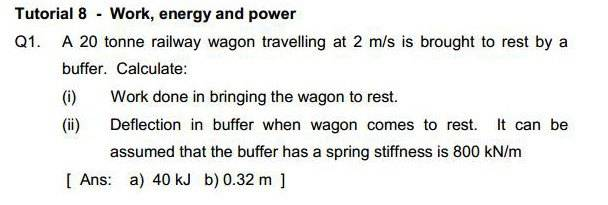# Work/Energy Question - Buffer

Hi, I've attached the question and answer below.

I've got (i) but I'm unsure how to achieve the answer for (ii).

I've tried using the linear motion equations and also a combination of the kinetic/potential energy questions but haven't had any luck as of yet...

Help is much appreciated, thanks!berkeman
Mentor
Hi, I've attached the question and answer below.

I've got (i) but I'm unsure how to achieve the answer for (ii).

I've tried using the linear motion equations and also a combination of the kinetic/potential energy questions but haven't had any luck as of yet...

Help is much appreciated, thanks!(In the future, please use the Homework Help Template that you are provided, and fill out each section. That makes it easier for us to help.)

What method did you use for (i)? Can you show us your calculations?

For (ii), Hint -- what is the equation for the energy stored in a spring?

(In the future, please use the Homework Help Template that you are provided, and fill out each section. That makes it easier for us to help.)

What method did you use for (i)? Can you show us your calculations?

For (ii), Hint -- what is the equation for the energy stored in a spring?

Hi, thanks, will do.

I used the kinetic energy formula 1/2mv^2 for the first answer.

I've been able to work it out using that formula, thanks, I wasn't aware of it previously.